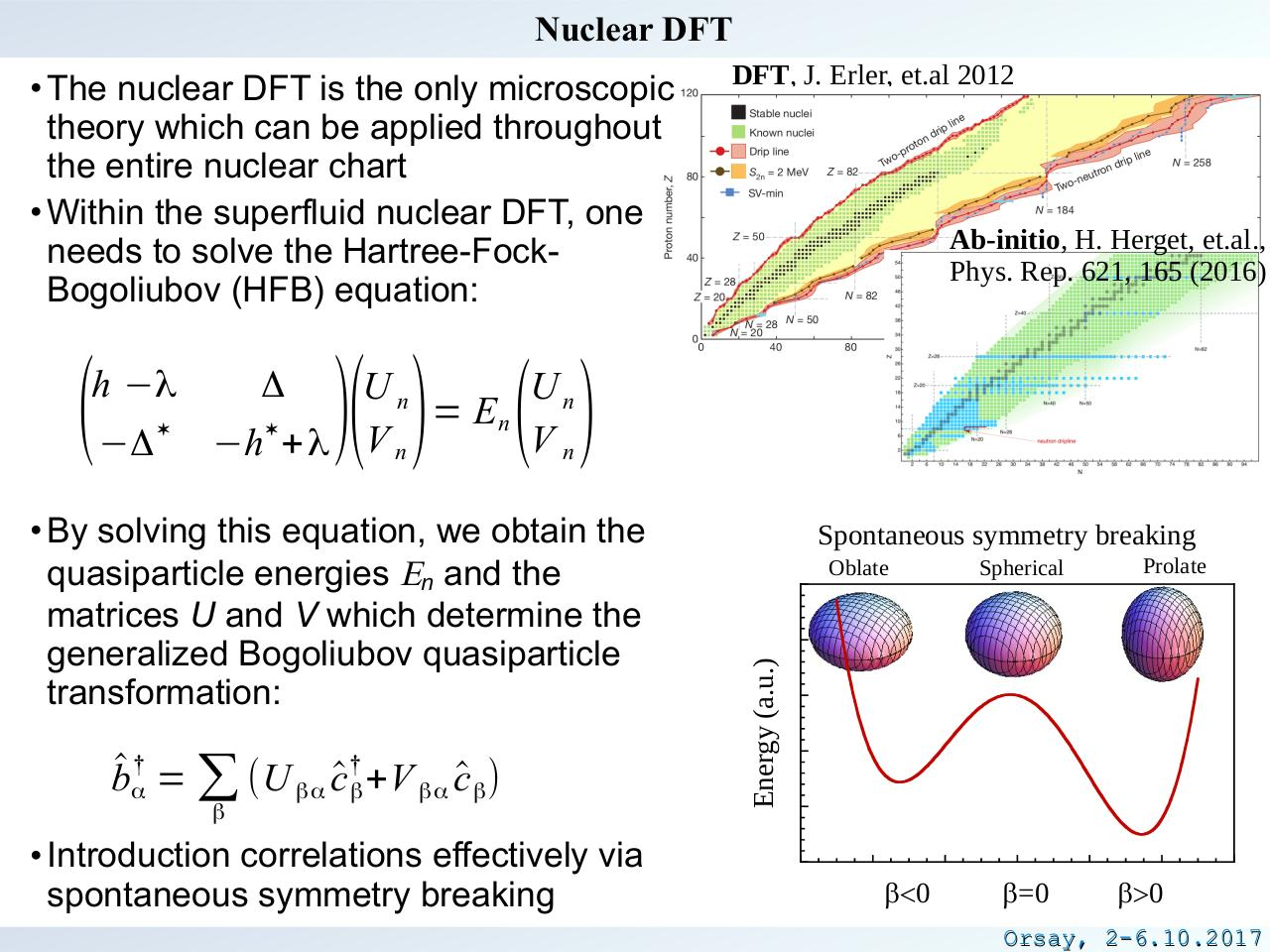# kortelainen.pdfPage 1 23421

#### Text preview

Nuclear DFT
• The nuclear DFT is the only microscopic
theory which can be applied throughout
the entire nuclear chart
• Within the superfluid nuclear DFT, one
needs to solve the Hartree-FockBogoliubov (HFB) equation:

−Δ

Δ

Ab-initio, H. Herget, et.al.,
Phys. Rep. 621, 165 (2016)

)( ) ( )

Un
Un
= En

Vn
−h + λ V n

• By solving this equation, we obtain the
quasiparticle energies En and the
matrices U and V which determine the
generalized Bogoliubov quasiparticle
transformation:

b^ α† = ∑ (U βα c^ †β +V βα c^ β )
β

• Introduction correlations effectively via
spontaneous symmetry breaking

Spontaneous symmetry breaking
Oblate

Spherical

Prolate

Energy (a.u.)

(

h −λ

DFT, J. Erler, et.al 2012

b&lt;0

b=0

b&gt;0

Orsay, 2-6.10.2017